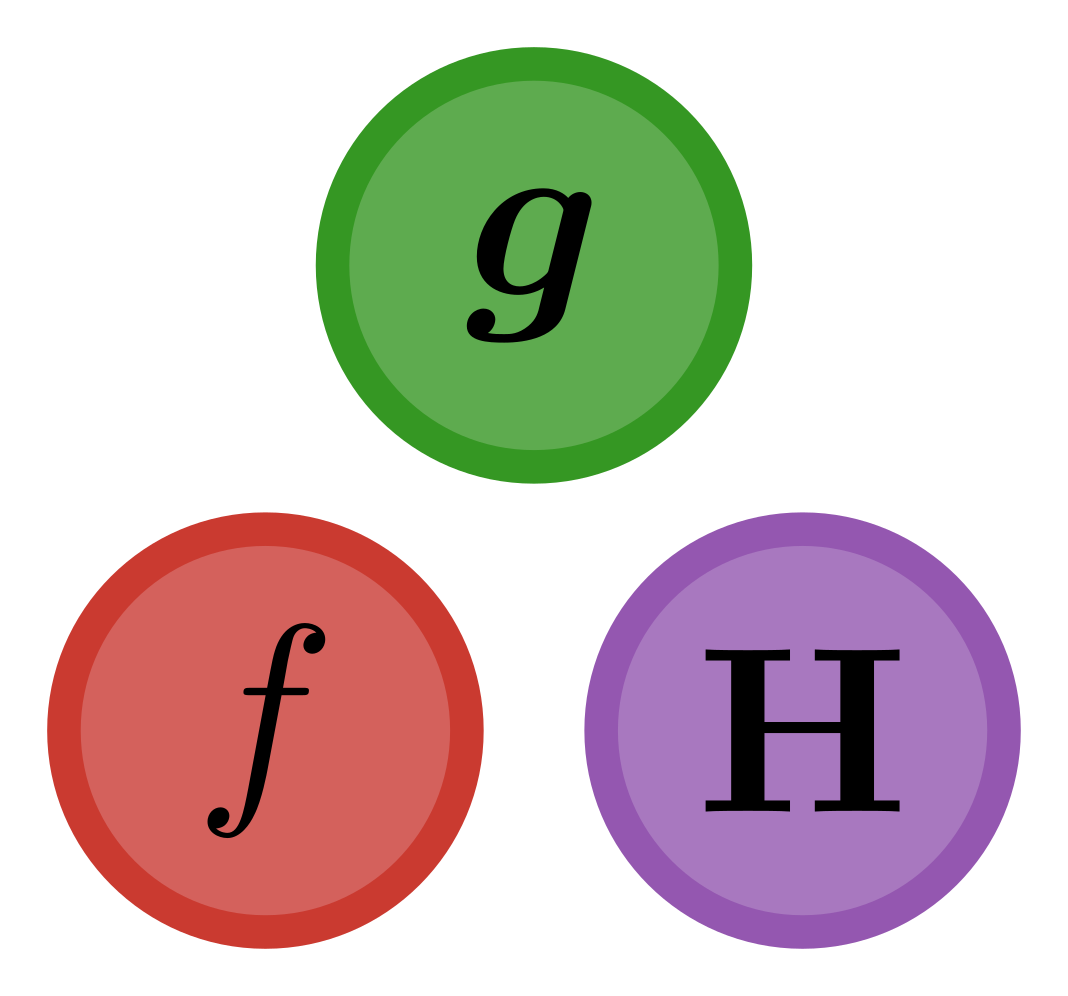## F1Method.jl

F-1 method
Author briochemc
Popularity
2 Stars
Updated Last
2 Years Ago
Started In
April 2019# F-1 algorithm

This package implements the F-1 algorithm described in Pasquier and Primeau (in preparation). It allows for efficient quasi-auto-differentiation of an objective function defined implicitly by the solution of a steady-state problem.

Consider a discretized system of nonlinear partial differential equations that takes the form

``````F(x,p) = 0
``````

where `x` is a column vector of the model state variables and `p` is a vector of parameters. The F-1 algorithm then allows for an efficient computation of both the gradient vector and the Hessian matrix of a generic objective function defined by

``````objective(p) = f(s(p),p)
``````

where `s(p)` is the steady-state solution of the system, i.e., such that `F(s(p),p) = 0` and where `f(x,p)` is for example a measure of the mismatch between observed state, parameters, and observations. Optimizing the model is then simply done by minimizing `objective(p)`. (See Pasquier and Primeau (in preparation), for more details.)

## Advantages of the F-1 algorithm

The F-1 algorithm is easy to use, gives accurate results, and is computationally fast:

• Easy — The F-1 algorithm basically just needs the user to provide a solver (for finding the steady-state), the mismatch function, `f`, the state function, `F`, and their derivatives, `∇ₓf` and `∇ₓF` w.r.t. the state `x`. (Note these derivatives can be computed numerically, via the ForwardDiff package for example.)
• Accurate — Thanks to ForwardDiff's nested dual numbers implementation, the accuracy of the gradient and Hessian, as computed by the F-1 algorithm, are close to machine precision.
• Fast — The F-1 algorithm is as fast as if you derived analytical formulas for every first and second derivatives and used those in the most efficient way. This is because the bottleneck of such computations is the number of matrix factorizations, and the F-1 algorithm only requires a single one. In comparison, standard autodifferentiation methods that take the steady-state solver as a black box would require order `m` or `m^2` factorizations, where `m` is the number of parameters.

## What's needed?

A requirement of the F-1 algorithm is that the Jacobian matrix `A = ∇ₓf` can be created, stored, and factorized.

To use the F-1 algorithm, the user must:

• Make sure that there is a suitable algorithm `alg` to solve the steady-state equation
• overload the `solve` function and the `SteadyStateProblem` constructor from DiffEqBase. (An example is given in the CI tests — see, e.g., the `test/simple_setup.jl` file.)
• Provide the derivatives of `f` and `F` with respect to the state, `x`.

## A concrete example

Make sure you have overloaded `solve` from DiffEqBase (an example of how to do this is given in the documentation). Once initial values for the state, `x`, and parameters, `p`, are chosen, simply initialize the required memory cache, `mem` via

```# Initialize the cache for storing reusable objects
mem = initialize_mem(F, ∇ₓf, ∇ₓF, x, p, alg; options...)```

wrap the functions into functions of `p` only via

```# Wrap the objective, gradient, and Hessian functions
objective(p) = F1Method.objective(f, F, ∇ₓF, mem, p, alg; options...)
hessian(p) = F1Method.hessian(f, F, ∇ₓf, ∇ₓF, mem, p, alg; options...)```

and compute the objective, gradient, or Hessian via either of

```objective(p)

hessian(p)```

That's it. You were told it was simple, weren't you? Now you can test how fast and accurate it is!

## Citing the software

If you use this package, or implement your own package based on the F-1 algorithm please cite us. If you use the F-1 algorithm, please cite Pasquier and Primeau (in prep.). If you also use this package directly, please cite it! (Use the Zenodo link or the CITATION.bib file, which contains a bibtex entry.)

### Required Packages

View all packages

### Used By Packages

No packages found.